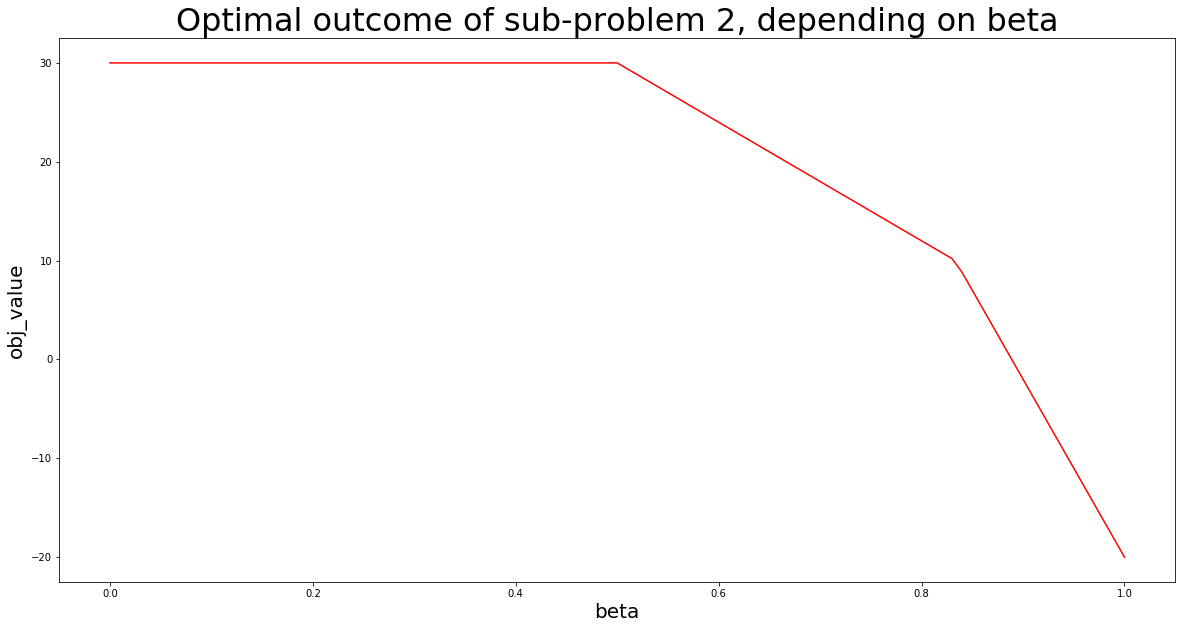# Multi-objective LP with sub-problem weightsIn my most recent post on linear programming I applied PuLP for solving below linear optimization problem, using two approaches. Approach #1 was based on solving a sub-problem with one objective only first, then adding the optimal outcome of that problem to a second sub-problem that considered objective two only. Approach #2 is based on combining all objectives into one overall objective function, combining single objectives with scalar weights.

In this post I want to show an alternative approach for solving below multi-objective linear optimization problem: I will use approach #1, but apply weights instead:

The approach I now use is to solve the problem in two steps, where a sub-problem with one objective only is solved first and its optimal outcome is added to a second sub-problem with only the the second objective as a constraint. This approach has been demonstrated by before, but this time I will apply a scalar weight-factor to that constraint, considering the optimal outcome of sub-problem with 0-100%.

Starting with the first objective, the first sub-problem to solve would be the following:

The optimal objective value to above sub-problem is 30.

After having solved the first sub-problem, the second objective would be considered by a second sub-problem adding the optimal outcome to above problem as a weighted constraint:

Here is the implementation in Python, using the PuLP module and applying a step-size of 0.01:

```# import PuLP for modelling and solving problems
import pulp

# import matplotlib.pyplot for visualization
import matplotlib.pyplot as plt

# import pandas and numpy for being able to store solutions in DataFrame
import numpy as np
import pandas as pd

# define step-size
stepSize = 0.01

# initialize empty DataFrame for storing optimization outcomes
solutionTable = pd.DataFrame(columns=["beta","x1_opt","x2_opt","obj_value"])

# declare optimization variables using PuLP and LpVariable
x1 = pulp.LpVariable("x1",lowBound=0)
x2 = pulp.LpVariable("x2",lowBound=0)

# model and solve sub-problem no. 1
linearProblem = pulp.LpProblem("First sub-problem",pulp.LpMaximize)
linearProblem += 2*x1 + 3*x2 # add objective no. 1
linearProblem += x1 + x2 <= 10 # add contraints from original problem statement
linearProblem += 2*x1 + x2 <= 15
solution = linearProblem.solve()

# store optimal outcome of sub-problem no. 1 into variable
optimalObj1 = pulp.value(linearProblem.objective)

# iterate through beta values from 0 to 1 with stepSize, and write PuLP solutions into solutionTable
for i in range(0,101,int(stepSize*100)):
# declare the problem again
linearProblem = pulp.LpProblem("Multi-objective linear maximization",pulp.LpMaximize)
# add the second objective as objective function to this sub-problem
linearProblem += 4*x1-2*x2
# add the constraints from original problem statement
linearProblem += x1 + x2 <= 10
linearProblem += 2*x1 + x2 <= 15
# add additional constraint at level beta, considering optimal outcome of sub-problem no. 1
linearProblem += 2*x1 + 3*x2 >= (i/100)*optimalObj1
# solve the problem
solution = linearProblem.solve()
# write solutions into DataFrame
solutionTable.loc[int(i/(stepSize*100))] = [i/100,
pulp.value(x1),
pulp.value(x2),
pulp.value(linearProblem.objective)]

# visualize optimization outcome, using matplotlib.pyplot
# -- set figure size
plt.figure(figsize=(20,10))
# -- create line plot
plt.plot(solutionTable["beta"],solutionTable["obj_value"],color="red")
plt.xlabel("beta",size=20)
plt.ylabel("obj_value",size=20)
plt.title("Optimal outcome of sub-problem 2, depending on beta",size=32)
# -- show plot
plt.show()
```

The results indicate that there is some range for beta where an increase in beta will not affect the optimal outcome of sub-problem 2, i.e. will not affect objective 2.

## 3 thoughts on “Multi-objective LP with sub-problem weights”

1.MitRa says:

thank you… what about nonlinear optimization? pulp doesnt support it 🙁

1.Linnart Felkl M.Sc. says:

Depends on what kind of optimization or problem we are talking about. Maybe check out nloptr for continuous problems. Also made a post on nloptr, however in R. Not Python.

2.Sal says:

Hey!
What if I wanted to plot the value of one objective function against the other instead of beta? How can I put the value of the OF that is being looped in the df?